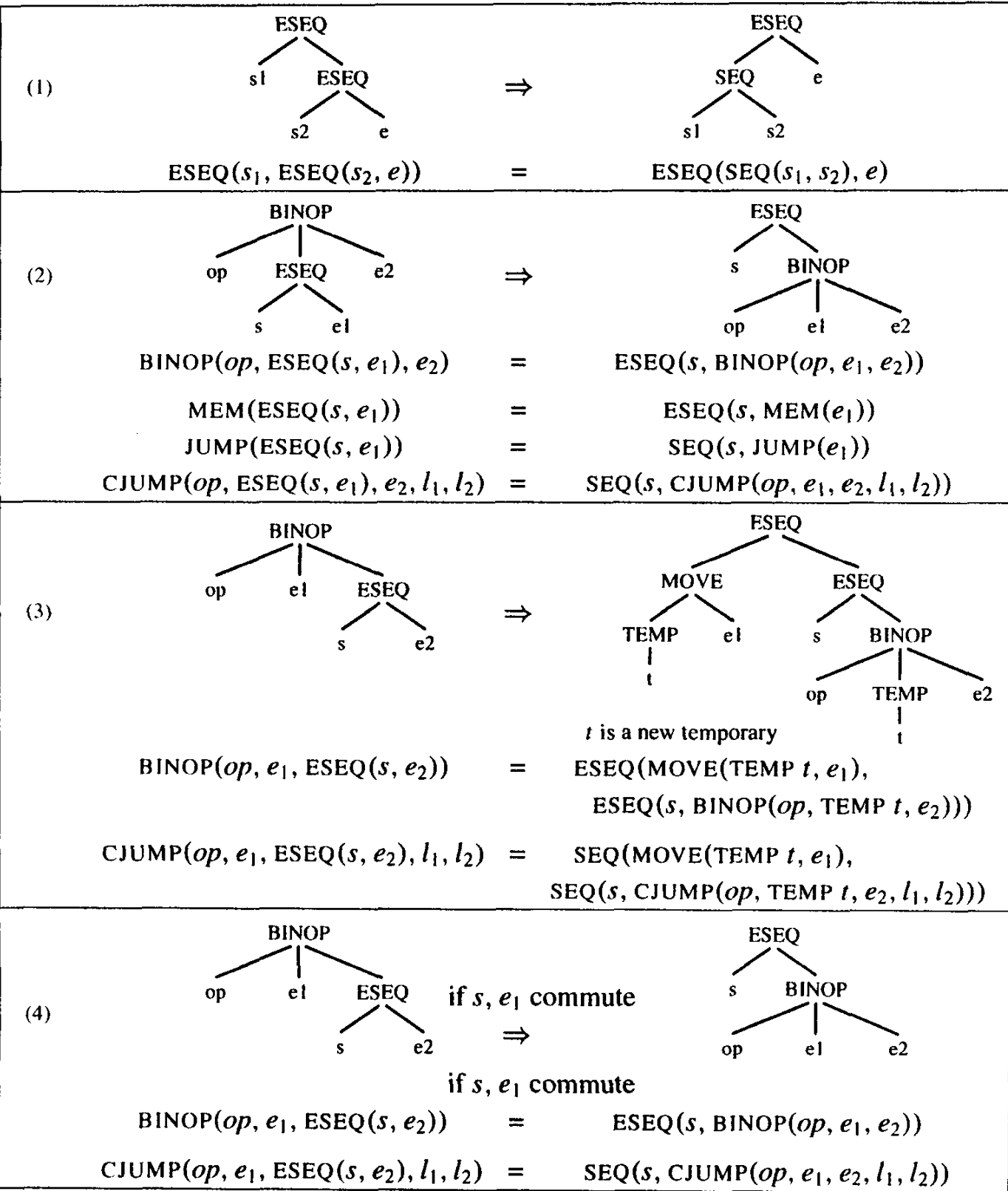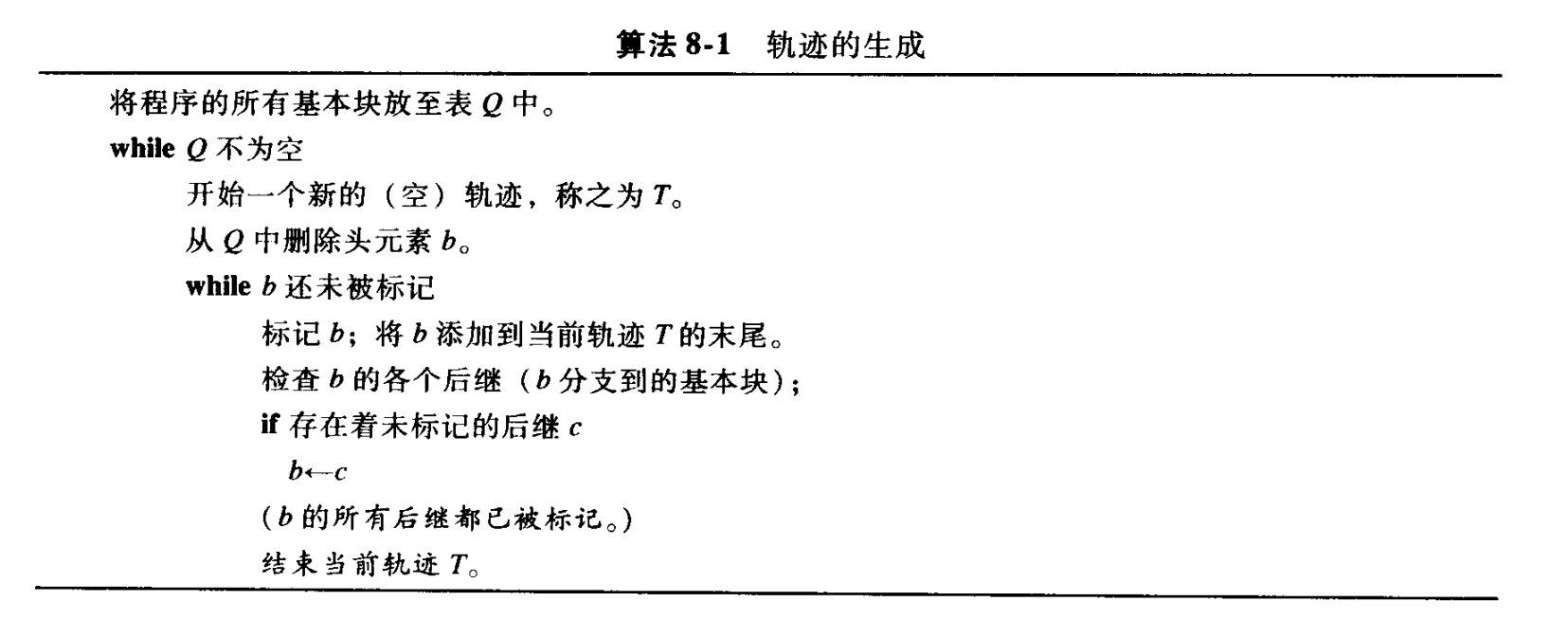# IR Generation

• 中间表示（intermediate representation, IR）

## 中间表示树 IR Trees

### 抽象语法表达式 $\mathrm{A}\mathrm{_}\mathrm{e}\mathrm{x}\mathrm{p}$

• $\mathrm{E}\mathrm{x}$：“表达式”，表示为 $\mathrm{T}\mathrm{r}\mathrm{_}\mathrm{e}\mathrm{x}\mathrm{p}$
• $\mathrm{N}\mathrm{x}$：“无结果语句”，表示为 $\mathrm{T}\mathrm{r}\mathrm{e}\mathrm{e}$ 语句
• $\mathrm{C}\mathrm{x}$：“条件语句”
static Tr_exp Tr_Ex(T_exp ex);
static Tr_exp Tr_Nx(T_stm nx);
static Tr_exp Tr_Cx(patchList trues, patchList falses, T_stm stm);


### 表达式 $\mathrm{T}\mathrm{_}\mathrm{e}\mathrm{x}\mathrm{p}$

• $\mathrm{C}\mathrm{O}\mathrm{N}\mathrm{S}\mathrm{T}\left(i\right)$：整型常数 $i$
• $\mathrm{N}\mathrm{A}\mathrm{M}\mathrm{E}\left(n\right)$：符号常数 $n$
• $\mathrm{T}\mathrm{E}\mathrm{M}\mathrm{P}\left(t\right)$：临时变量 $t$
• $\mathrm{B}\mathrm{I}\mathrm{N}\mathrm{O}\mathrm{P}\left(o,{e}_{1},{e}_{2}\right)$：对操作数 ${e}_{1}$${e}_{2}$ 施加二元操作符 $o$ 表示的操作
• $\mathrm{M}\mathrm{E}\mathrm{M}\left(e\right)$：开始于存储地址 $e$ 的 wordSize 个字节的内容
• $\mathrm{C}\mathrm{A}\mathrm{L}\mathrm{L}\left(f,l\right)$：过程调用，以参数表 $l$ 调用函数 $f$
• $\mathrm{E}\mathrm{S}\mathrm{E}\mathrm{Q}\left(s,e\right)$：先计算语句 $s$ 以形成其副作用，然后计算 $e$ 作为此表达式的结果
• ：计算 $e$ 并将结果送入临时单元 $t$
• $\mathrm{M}\mathrm{O}\mathrm{V}\mathrm{E}\left(\mathrm{M}\mathrm{E}\mathrm{M}\left({e}_{1}\right),{e}_{2}\right)$：计算 ${e}_{1}$，由它生成地址 $a$，然后计算 ${e}_{2}$，并将计算结果存储在从地址 $a$ 开始的 wordSize 个字节的存储单位中
• $\mathrm{E}\mathrm{X}\mathrm{P}\left(e\right)$：计算 $e$ 但忽略结果
• $\mathrm{J}\mathrm{U}\mathrm{M}\mathrm{P}\left(e,labs\right)$
• $\mathrm{C}\mathrm{J}\mathrm{U}\mathrm{M}\mathrm{P}\left(o,{e}_{1},{e}_{2},t,f\right)$：依次计算 ${e}_{1}$${e}_{2}$，生成值 $a$$b$，然后用关系操作符 $o$ 比较 $a$$b$；如果结果为 true，则跳转到 $t$，否则跳转到 $f$
• 关系操作符 $o$ 可以是 LT、GT、LE、GE、EQ、NE...
• $\mathrm{S}\mathrm{E}\mathrm{Q}\left({s}_{1},{s}_{2}\right)$：语句 ${s}_{1}$ 之后跟随语句 ${s}_{2}$
• $\mathrm{L}\mathrm{A}\mathrm{B}\mathrm{E}\mathrm{L}\left(n\right)$：定义名字 $n$ 的常数值为当前机器代码的地址

## 基本块和轨迹

Tree 语言表示的程序和机器语言程序之间存在如下不匹配的情况：

• 在表达式中使用 $\mathrm{C}\mathrm{J}\mathrm{U}\mathrm{M}\mathrm{P}$ 会使得转移与机器语言的规则不同
• 机器语言的转移指令在条件为假时是下降至下一条指令
• 在表达式中使用 $\mathrm{E}\mathrm{S}\mathrm{E}\mathrm{Q}$ 会使得子树的不同计算顺序产生不同结果
• 在表达式中使用 $\mathrm{C}\mathrm{A}\mathrm{L}\mathrm{L}$ 也会使得子树的不同计算顺序产生不同结果
• 在一个 $\mathrm{C}\mathrm{A}\mathrm{L}\mathrm{L}$ 结点的参数表达式中使用另一个 $\mathrm{C}\mathrm{A}\mathrm{L}\mathrm{L}$ 结点会出问题

1. 将一棵树重写成一列不含 $\mathrm{S}\mathrm{E}\mathrm{Q}$$\mathrm{E}\mathrm{S}\mathrm{E}\mathrm{Q}$ 结点的 规范树
2. 将一列树分组组合成其内不含转移和标号的 基本块 集合
3. 对基本块排序并形成一组 轨迹，轨迹中每一个 $\mathrm{C}\mathrm{J}\mathrm{U}\mathrm{M}\mathrm{P}$ 之后都直接跟随它的 false 标号

### 规范树 canonical tree

• 定义具有以下属性的树为规范树
1. $\mathrm{S}\mathrm{E}\mathrm{Q}$$\mathrm{E}\mathrm{S}\mathrm{E}\mathrm{Q}$
2. 每一个 $CALL$ 的父亲不是 $\mathrm{E}\mathrm{X}\mathrm{P}\left(\dots \right)$，就是

#### ESEQ 的转换#### 将 CALL 移到顶层

$\mathrm{C}\mathrm{A}\mathrm{L}\mathrm{L}$ 的实现是将它的结果返回到同一个规定的返回值寄存器 $\mathrm{T}\mathrm{E}\mathrm{M}\mathrm{P}\left(\mathrm{R}\mathrm{V}\right)$ 中，所以像是 $\mathrm{B}\mathrm{I}\mathrm{N}\mathrm{O}\mathrm{P}\left(\mathrm{P}\mathrm{L}\mathrm{U}\mathrm{S},\mathrm{C}\mathrm{A}\mathrm{L}\mathrm{L}\left(\dots \right),\mathrm{C}\mathrm{A}\mathrm{L}\mathrm{L}\left(\dots \right)\right)$ 的语句都存在冲突，但是可以使用 重写规则 解决

• 对于 $\mathrm{C}\mathrm{A}\mathrm{L}\mathrm{L}\left(fun,args\right)$，替换为
• 对于 $\mathrm{M}\mathrm{O}\mathrm{V}\mathrm{E}\left(\mathrm{T}\mathrm{E}\mathrm{M}\mathrm{P}{t}_{new},\mathrm{C}\mathrm{A}\mathrm{L}\mathrm{L}\left(f,args\right)\right)$，不执行替换
• 对于 $\mathrm{E}\mathrm{X}\mathrm{P}\left(\mathrm{C}\mathrm{A}\mathrm{L}\mathrm{L}\left(f,args\right)\right)$，不执行替换

#### 线性语句表

$\mathrm{S}\mathrm{E}\mathrm{Q}\left({s}_{1},\mathrm{S}\mathrm{E}\mathrm{Q}\left({s}_{2},\dots ,\mathrm{S}\mathrm{E}\mathrm{Q}\left({s}_{n-1},{s}_{n}\right)\dots \right)\right)$

${s}_{1},{s}_{2},\dots ,{s}_{n-1},{s}_{n}$

### 基本块 basic block

• 基本块是语句组成的一个序列，需要满足以下条件
1. 第一个语句是一个 $\mathrm{L}\mathrm{A}\mathrm{B}\mathrm{E}\mathrm{L}$
2. 最后一个语句是 $\mathrm{J}\mathrm{U}\mathrm{M}\mathrm{P}$ 或者 $\mathrm{C}\mathrm{J}\mathrm{U}\mathrm{M}\mathrm{P}$
3. 没有其他的 $\mathrm{L}\mathrm{A}\mathrm{B}\mathrm{E}\mathrm{L}$$\mathrm{J}\mathrm{U}\mathrm{M}\mathrm{P}$ 或者 $\mathrm{C}\mathrm{J}\mathrm{U}\mathrm{M}\mathrm{P}$
• 可以按任意顺序安排基本块，并且程序执行的结果仍是相同的

### 轨迹 trace

• 轨迹是在程序执行期间可能连贯执行的语句序列